## Goals for today

1. Continue to practice data visualization with ggplot2

2. Continue to practice data transformation with dplyr

3. Integrate 1) and 2) to explore the gapminder dataset

## General instructions

• Today, we’ll combine the powerful data transformation tools in dplyr and the data visualization tools in ggplot2 to continue our visual exploration of global trends in public health and economics compiled by the Gapminder project.

• To start, first open a new RMarkdown file in your course repo, set the output format to github_document, save it in your lab folder as lab4.Rmd, and work in this RMarkdown file for the rest of this lab.

• Now that you have learned how to subset data using different functions in dplyr, we will work with the full gapminder dataset provided in the R package dslabs. Let’s start by installing the dslabs package if you don’t have it installed already. Then, we need to load it with the library() function. We also need to load the tidyverse package because it contains ggplot.

library(tidyverse)
library(dslabs) #install.packages("dslabs")

# After you have loaded the dslabs package, you can access the data stored in gapminder. Let's look at the top 5 lines
gapminder %>% as_tibble() %>%
head(5)
## # A tibble: 5 x 9
##   country    year infant_mortality life_expectancy fertility population      gdp
##   <fct>     <int>            <dbl>           <dbl>     <dbl>      <dbl>    <dbl>
## 1 Albania    1960            115.             62.9      6.19    1636054 NA
## 2 Algeria    1960            148.             47.5      7.65   11124892  1.38e10
## 3 Angola     1960            208              36.0      7.32    5270844 NA
## 4 Antigua …  1960             NA              63.0      4.43      54681 NA
## 5 Argentina  1960             59.9            65.4      3.11   20619075  1.08e11
## # … with 2 more variables: continent <fct>, region <fct>

• As a reminder, to get familar with this dataset, you might want to use functions like View(), dim(), colnames() , and ?. You will see that the dataset includes the following variables:

• country
• year
• infant_mortality (infant deaths per 1000)
• life_expectancy
• fertility (average number of children per woman)
• population (per country)
• gpd (per country)
• continent
• region (geographical region)

## Exercise 1: Use data transformation and visualization to answer the following questions in breakout rooms (45 min)

The mission of the Gapminder Project is to “fight devastating ignorance with a fact-based worldview everyone can understand”. Per their own description, Gapminder identifies systematic misconceptions about important global trends and proportions and uses reliable data to develop easy to understand teaching materials to rid people of their misconceptions.

Several of the questions posted below have been borrowed from their ignorance test

You may first answer these questions based on your intuition, and then use the gapminder dataset to verify if your intuition is correct, either with a summary table of the relevant statistics or with a visualization (ideally both!).

We provide one possible solution for each question, but we highly recommend that you don’t look at them unless you are really stuck.

### Question 1: Which five countries had the largest population size in 2015 (the most recent year for which population sizes are included in this dataset), and how has the population sizes in those countries changes since 1960?

One possible solution
click to expand
# Extract a vector with the 5 countries with the largest population size
top5_countries <- gapminder %>%
filter(year == 2015) %>%
arrange(-population) %>%
select(country) %>%
head(5) %>%
pull()

gapminder %>%
filter(country %in% top5_countries) %>%
ggplot() +
geom_line(mapping = aes(x = year, y = population, color = country))
## Warning: Removed 5 row(s) containing missing values (geom_path).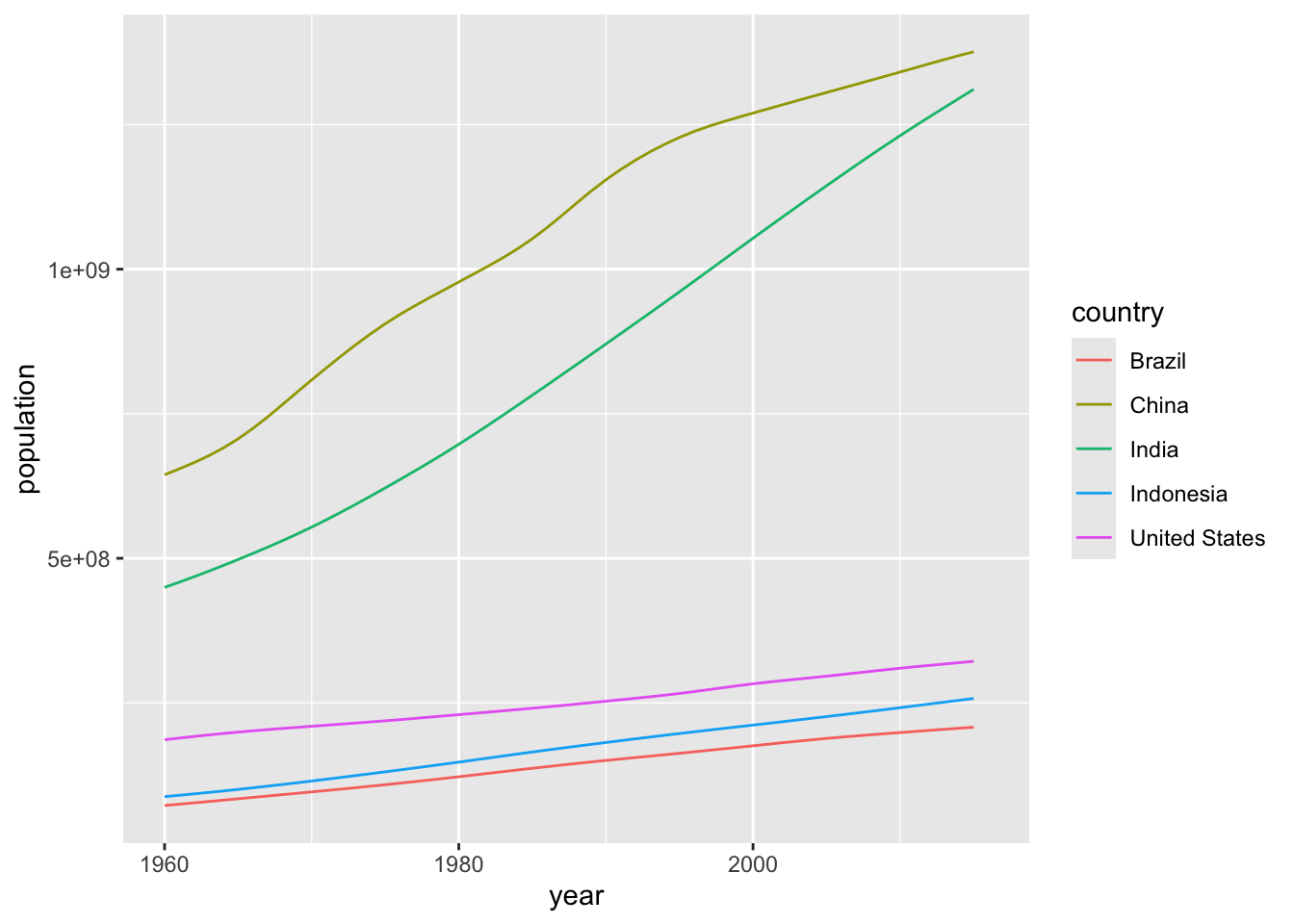### Question 2. Rank the following countries in infant mortality rate in 2015.

Turkey, Poland, South Korea, Russia, Vietnam, South Africa

One possible solution
click to expand
gapminder %>%
filter(year==2015, country %in% c("Turkey", "Poland", "South Korea", "Russia", "Vietnam", "South Africa")) %>%
arrange(infant_mortality) %>%
select(country, infant_mortality) %>%
knitr::kable()
country infant_mortality
South Korea 2.9
Poland 4.5
Russia 8.2
Turkey 11.6
Vietnam 17.3
South Africa 33.6

### Question 3. What is the general relationship between per-capita GDP and fertility rate?

A. Positive relationship
B. Negetive relationship
C. No relationship

Hint: use the data from 2000

One possible solution
click to expand
gapminder %>%
filter(year==2000) %>%
ggplot(aes(y=fertility, x=gdp/population)) +
geom_point() +
geom_smooth(se=F, method = "lm")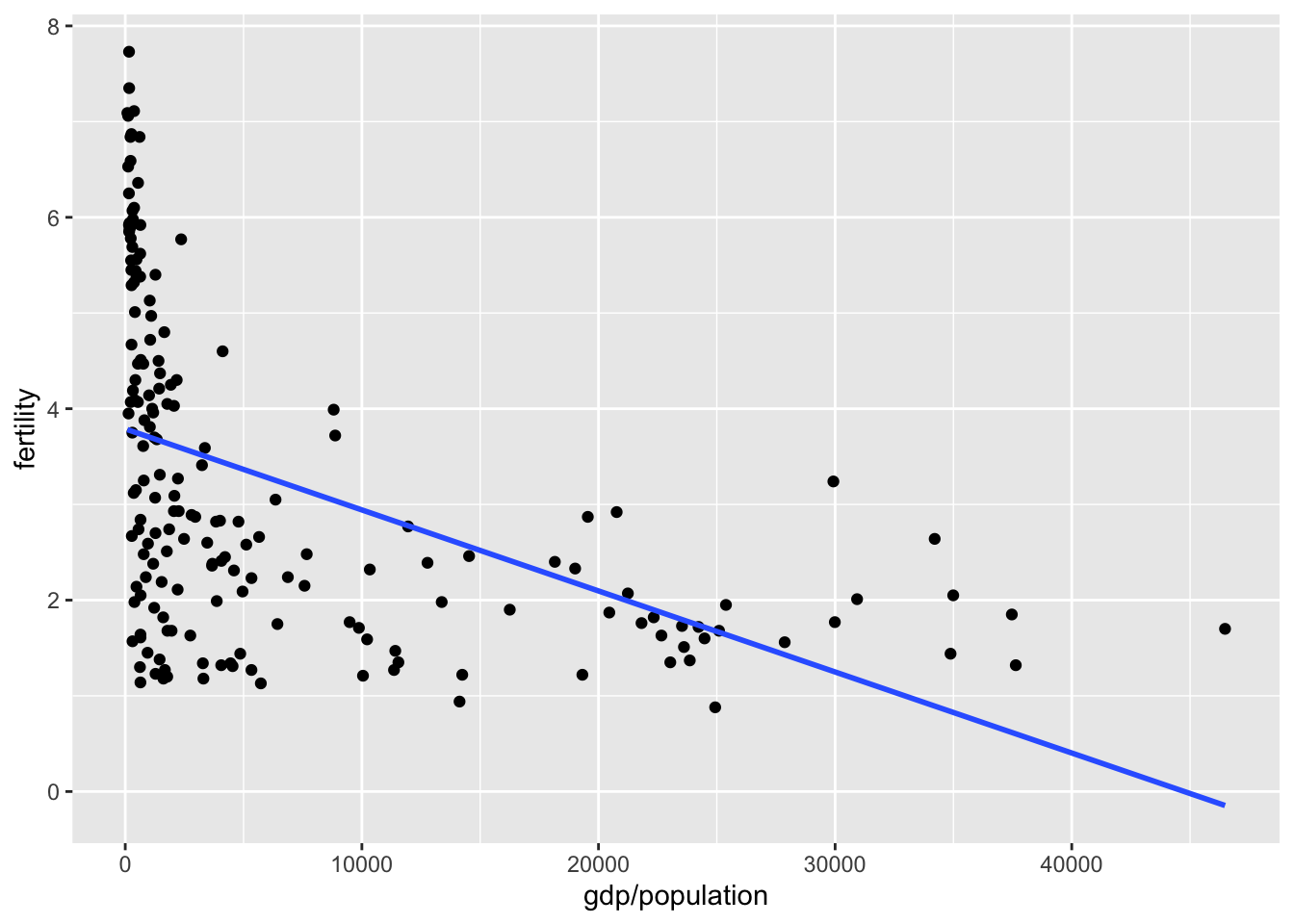### Question 4. If you break down the relationship between per-capita GDP and fertility rate by continent, which continent (or regions) stands out as an outlier? (Bonus question: why might this be?)

A. Africa
B. Asia
C. Europe

Hint: use the data from 2000

One possible solution
click to expand
gapminder %>%
filter(year==2000) %>%
ggplot(aes(y=fertility, x=gdp/population, color=continent)) +
geom_point() +
geom_smooth(se=F, method = "lm") +
facet_wrap(~continent, scales = "free_y")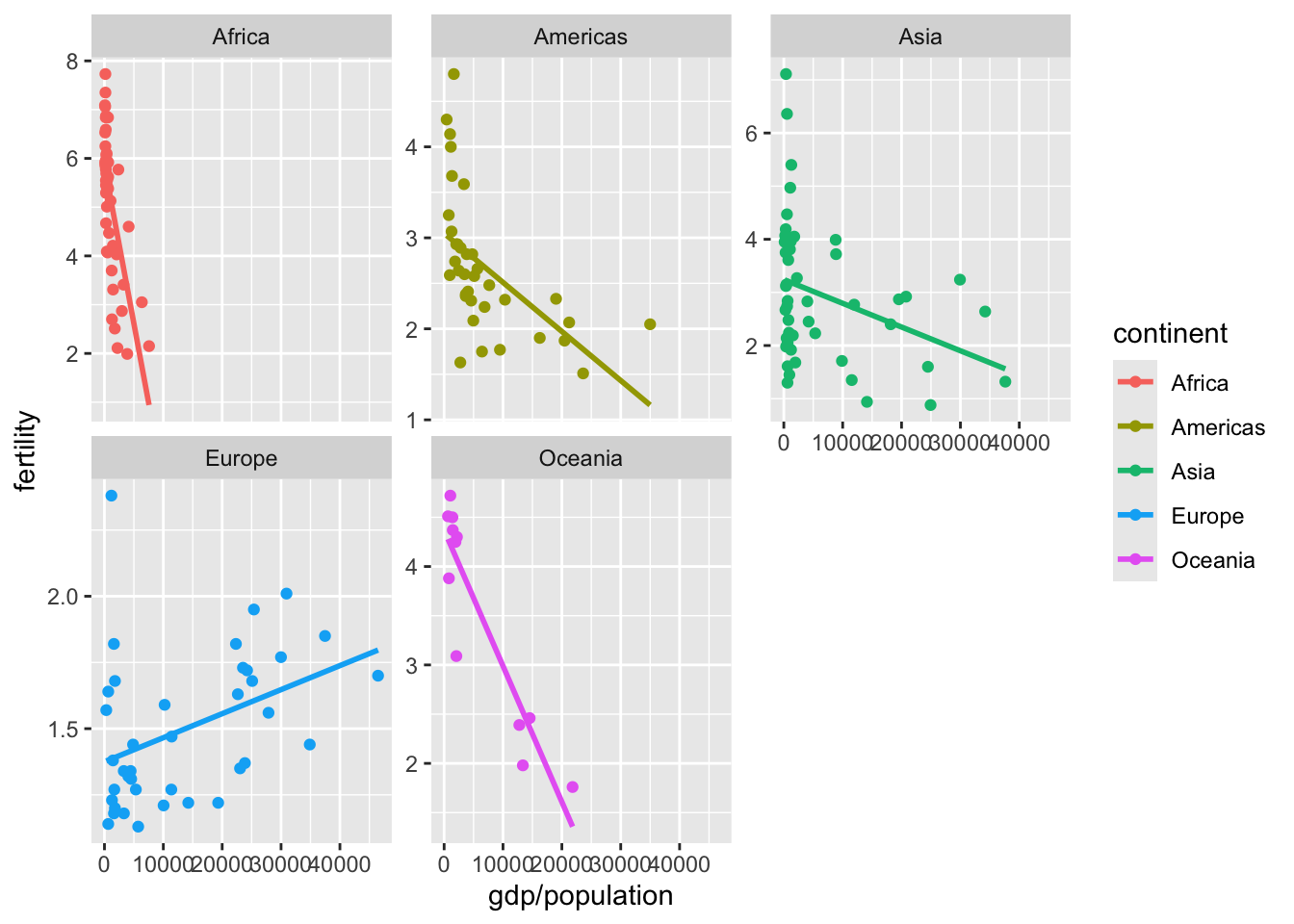eu_2000 <- gapminder %>%
filter(year==2000, continent == "Europe")
eu_2000 %>%
filter(fertility > 1.5, gdp/population > 20000) %>%
ggplot(aes(y=fertility, x=gdp/population, color=region)) +
ggrepel::geom_label_repel(aes(label=country)) +
geom_point(data=eu_2000)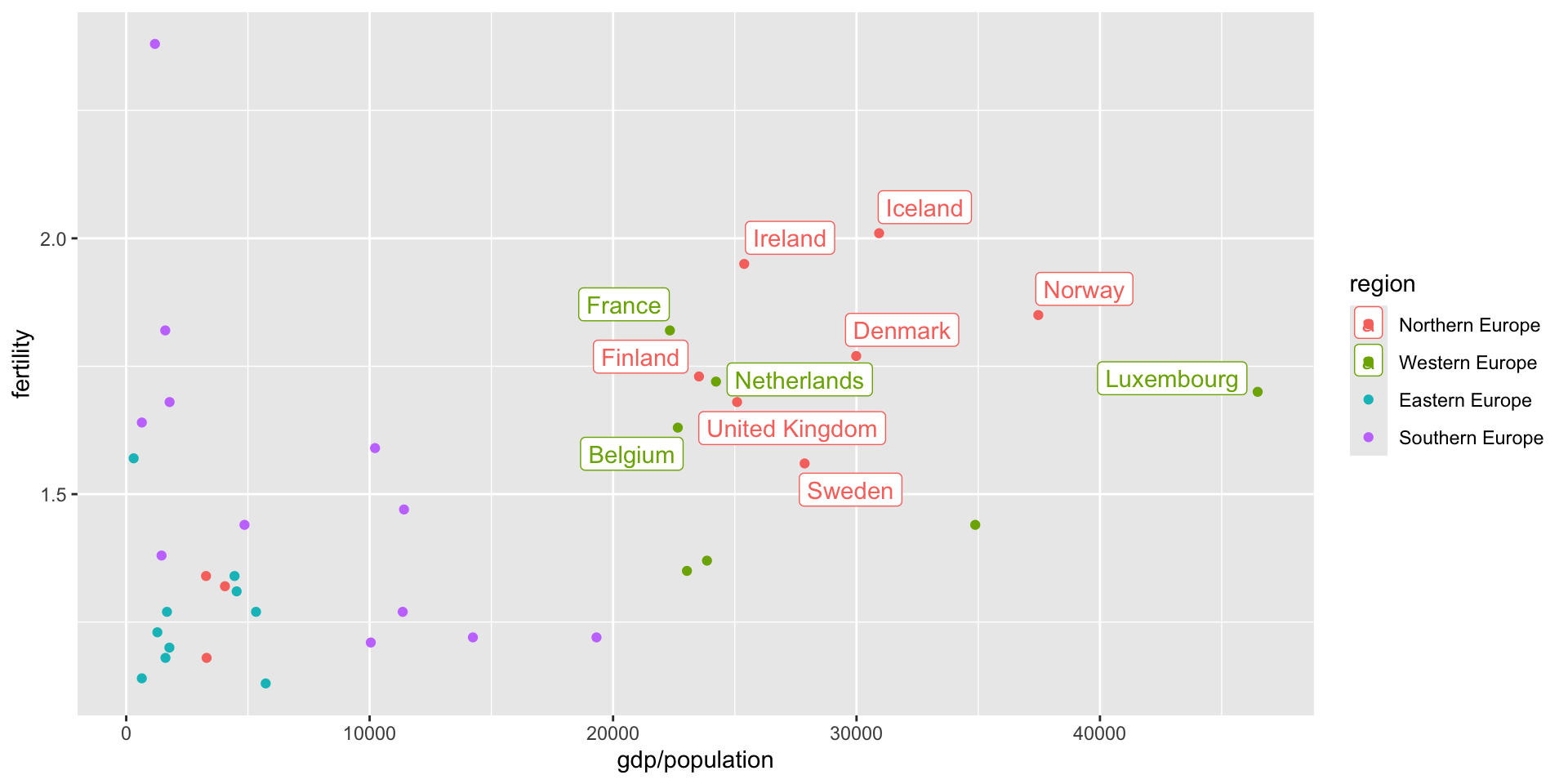### Question 5. There are roughly seven billion people in the world today. Which map shows where people live? (Each figure represents 1 billion people.)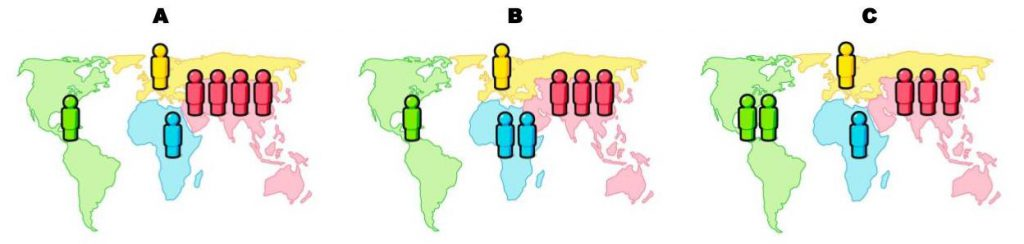Hint: use the data from 2015

One possible solution
click to expand
gapminder %>%
filter(year==2015) %>%
group_by(continent) %>%
summarize(population_in_billion=sum(population)/10^9) %>%
ggplot(aes(x=continent, y=population_in_billion)) +
geom_col()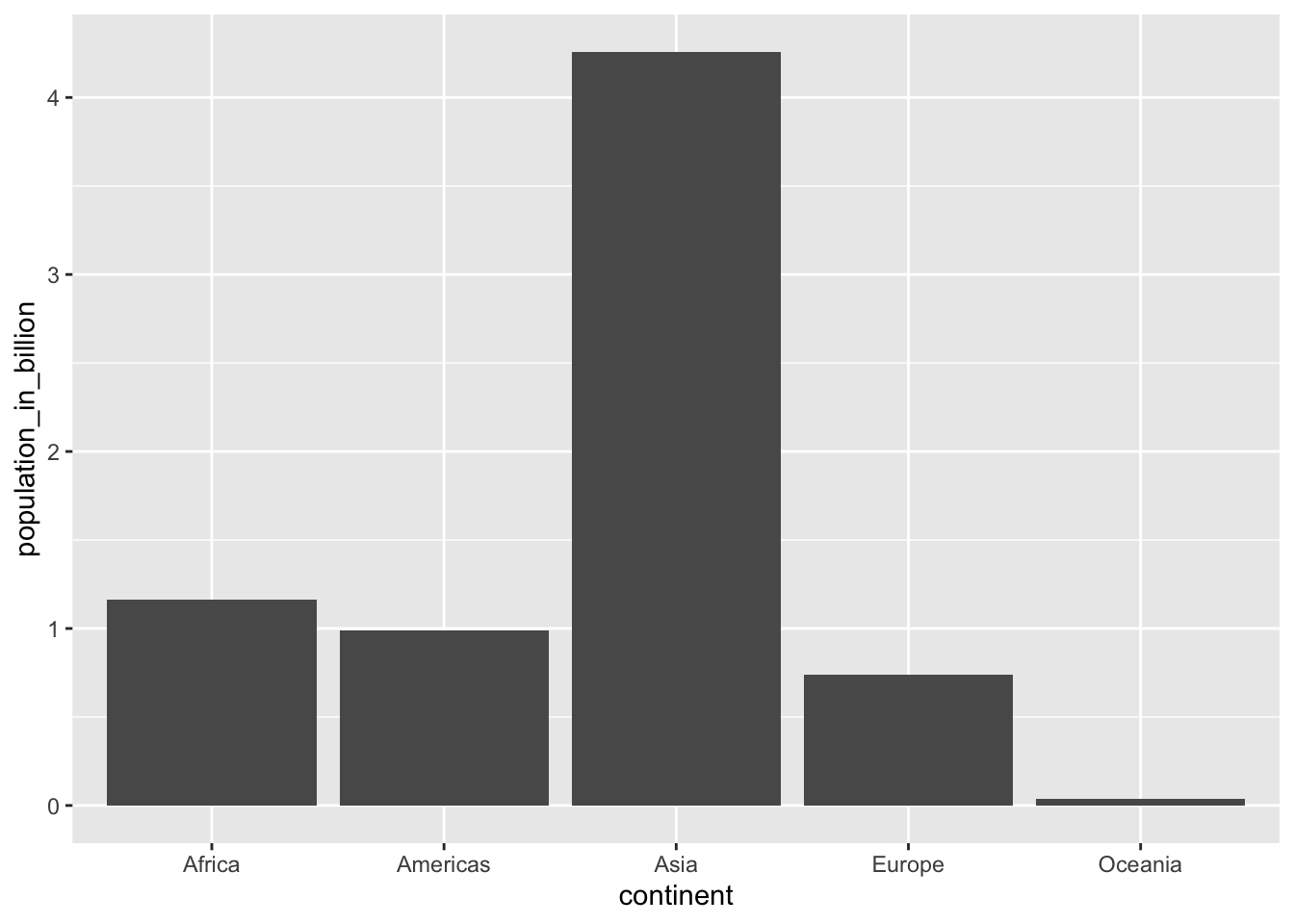### Question 6. What is the overall life expectancy for the world population (i.e. global average)?

A. 50 years
B. 60 years
C. 70 years

Hint: use the data from 2015

One possible solution
click to expand
gapminder %>%
filter(year==2015) %>%
summarize(life_expectancy=sum(life_expectancy*population)/sum(population))
##   life_expectancy
## 1         72.2457

### Question 7. What is the gap in life expectancy between Europe and Africa?

A. 5 years
B. 15 years
C. 25 years

Hint: use the data from 2015

One possible solution
click to expand
gapminder %>%
filter(year==2015) %>%
group_by(continent) %>%
summarize(life_expectancy=sum(life_expectancy*population)/sum(population)) %>%
ggplot(aes(x=continent, y=life_expectancy)) +
geom_col()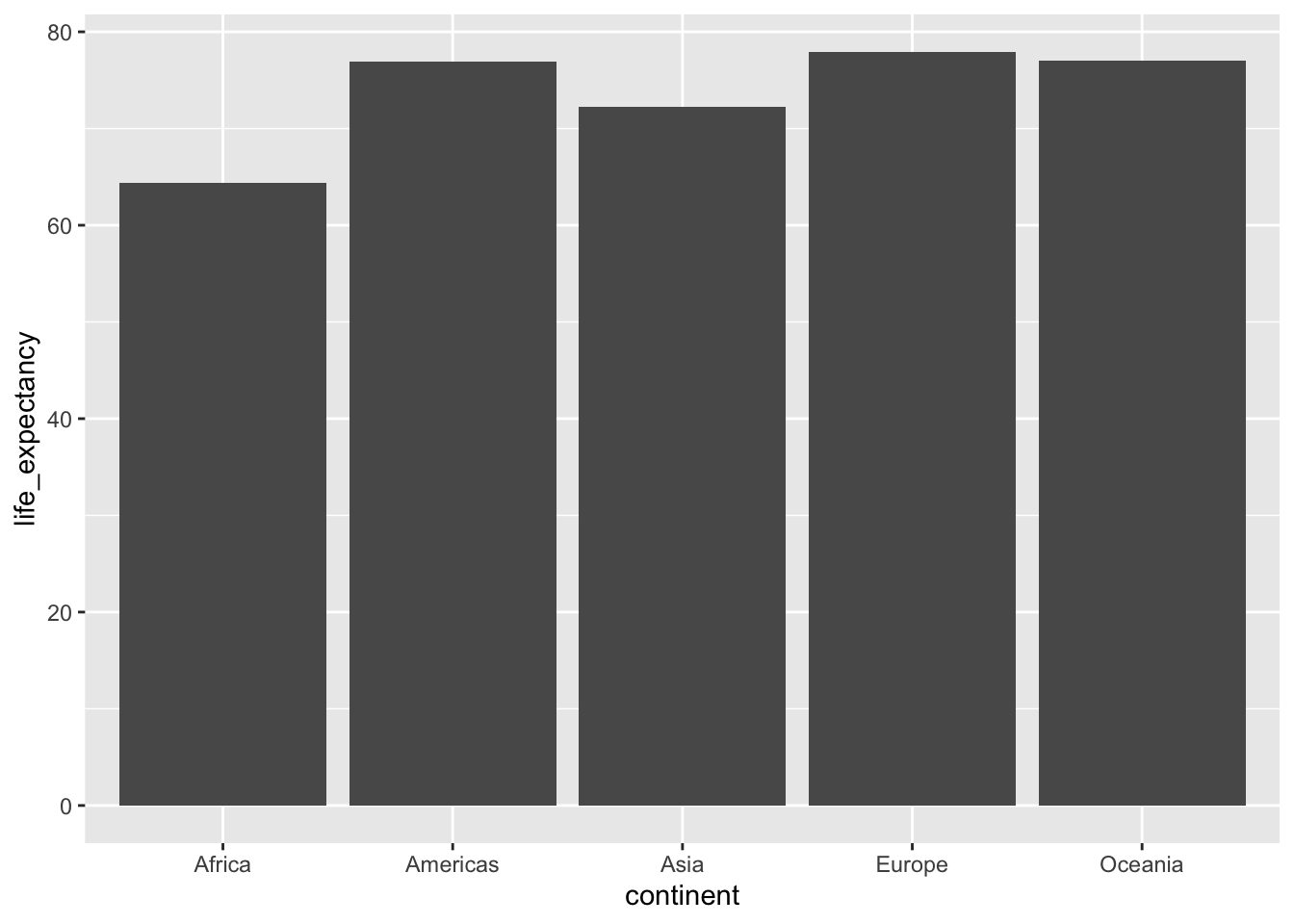gapminder %>%
filter(year==2015) %>%
ggplot(aes(x=continent, y=life_expectancy)) +
geom_jitter(aes(color=continent),height = 0) +
geom_boxplot(alpha=0, outlier.alpha = 0)## Recap (10 minutes)

Share your findings, challenges, and questions with the class.

## Exercise 2: Use data transformation and visualization to explore the following open-ended question in breakout rooms (40 min)

This question is borrowed from the excellent Chapter 9 in Rafael A. Irizarry’s Introduction to Data Science book

### Explore how much the gap in infant mortality, life expectancy, and per capita GDP between Western countries and the rest of the world have changed from 1960 to 2010.

Suggestions:

• Visualizing the entire time series and taking certain snapshots of time (e.g. one data point every decade) can both be useful approaches.

• The range in per capita GDP can be very high, with most countries having low values but a few countries having very high values, so a log transformation may be useful.

• You can try different definitions of “Western countries” and the “rest of the world”.

• You can also analyze different subgroups within the broad categorizations of “Western countries” and the “rest of the world” separately.

• Try to explore different geometric objects. Line plot, scatter plot, density plot, box plot, bar plot, and others can all be useful.

Some ideas if you are really stuck
click to expand
years <- c(1960, 1970, 1980, 1990, 2000, 2010)
continents <- c("Europe", "Asia")
gapminder %>%
filter(year %in% years & continent %in% continents) %>%
ggplot(aes(log(gdp/population), life_expectancy, col = continent)) +
geom_point() +
facet_wrap(~year) 
## Warning: Removed 148 rows containing missing values (geom_point).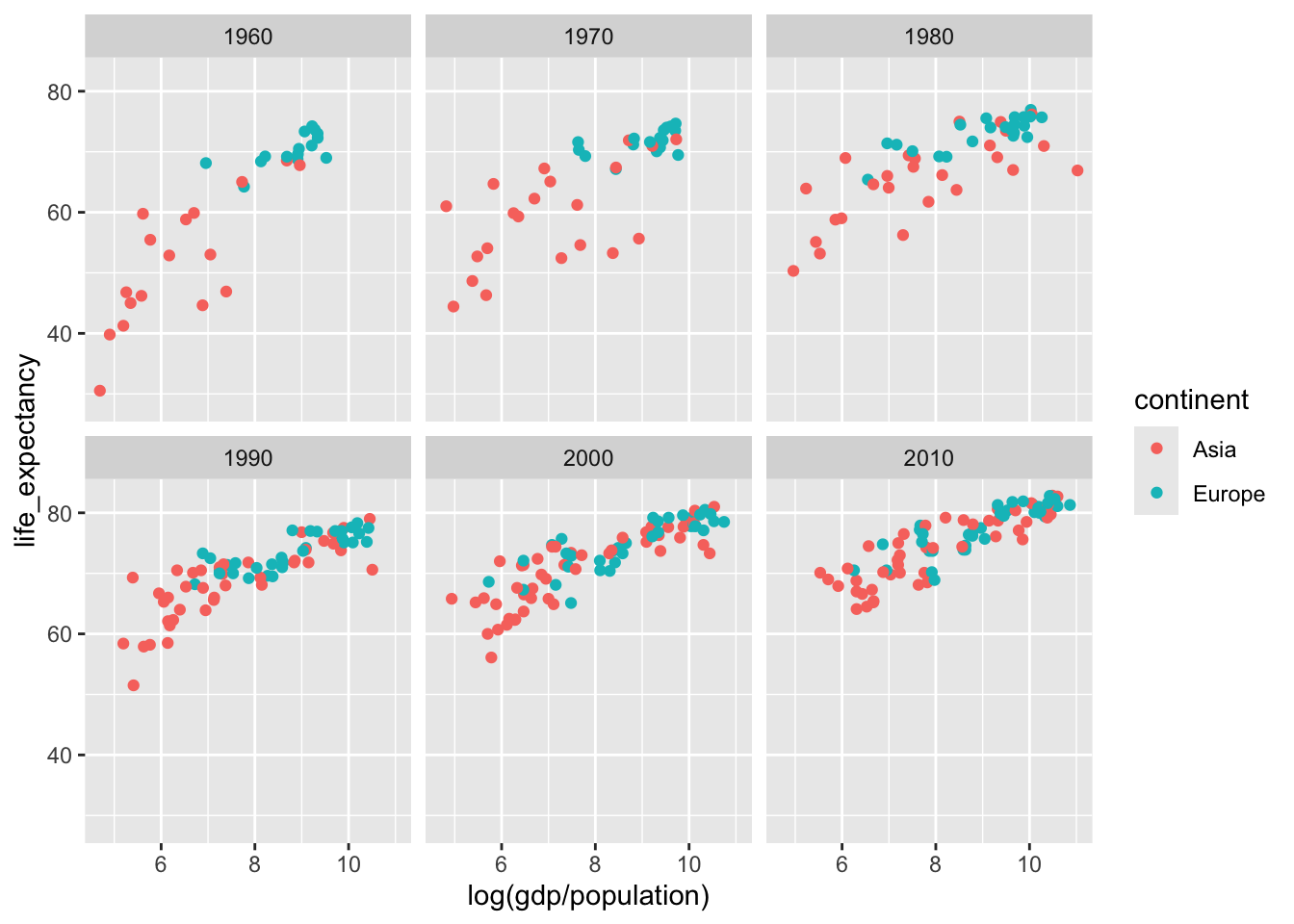gapminder %>%
filter(continent %in% continents) %>%
ggplot(aes(x=year, y=life_expectancy, group=country)) +
geom_line()+
facet_wrap(~continent)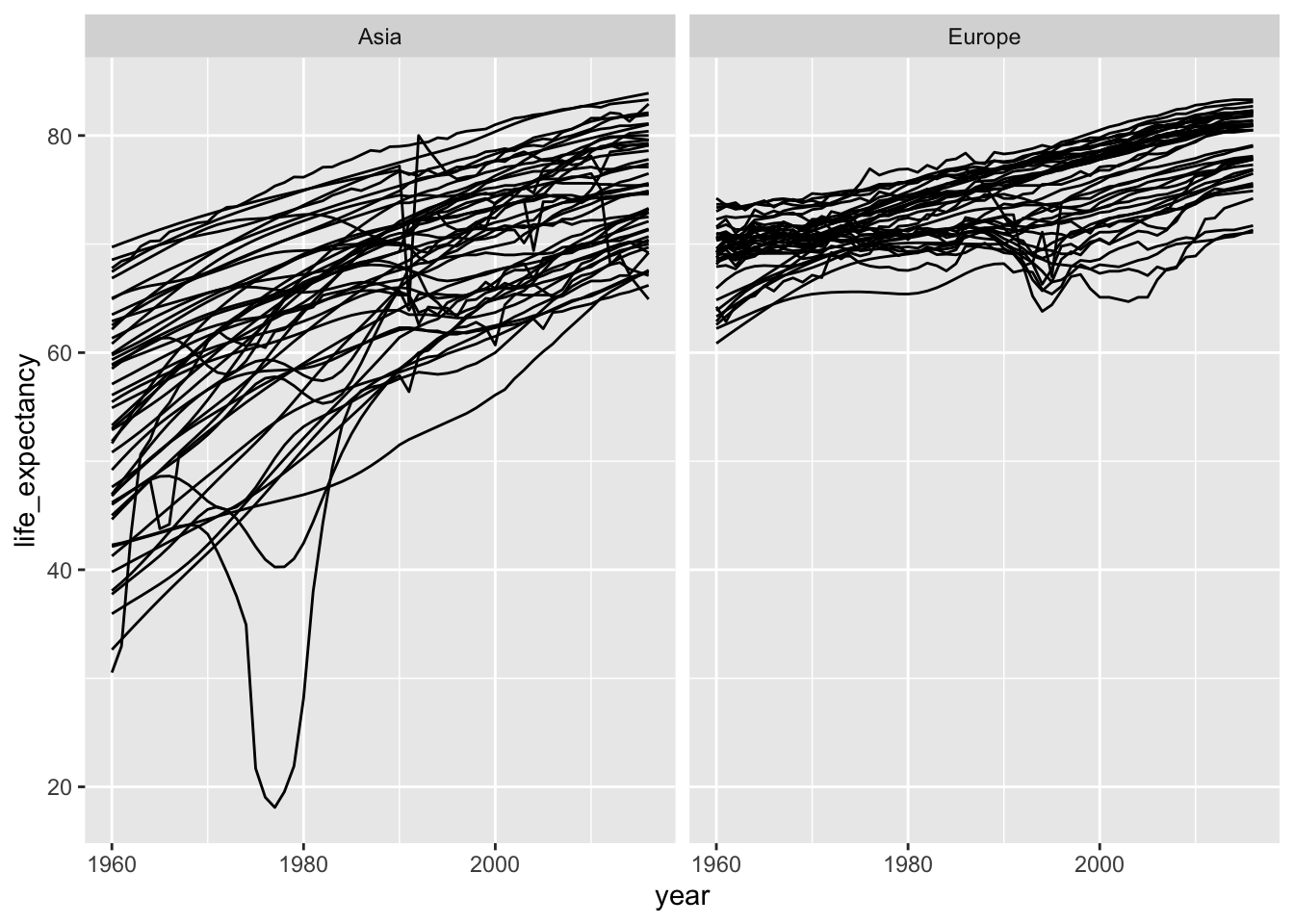gapminder %>%
filter(year %in% c(1960, 2010)) %>%
ggplot(aes(x=life_expectancy, fill=continent)) +
geom_density(alpha=0.5)+
facet_wrap(~year, nrow=2)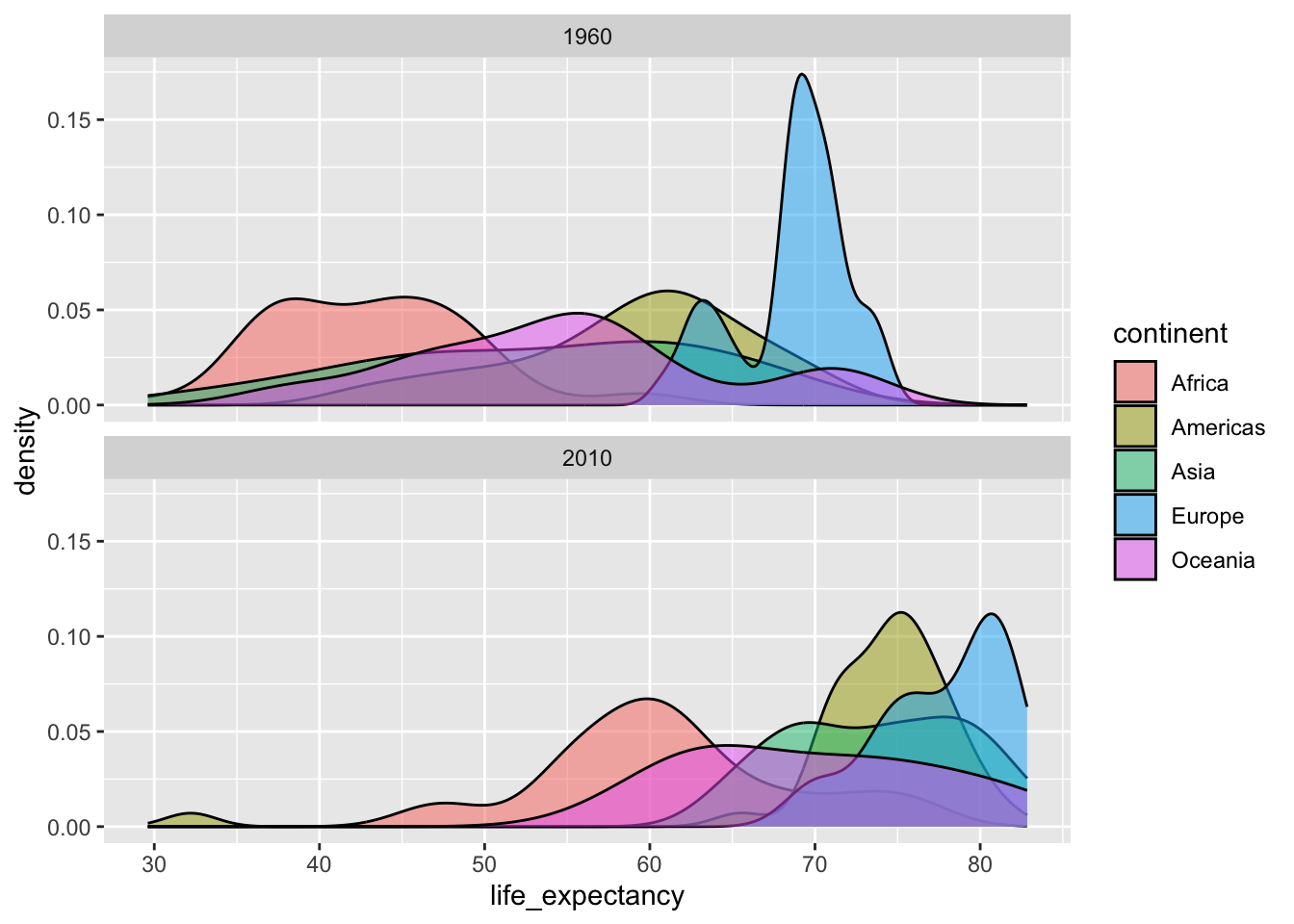gapminder %>%
filter(year %in% c(1960, 2010)) %>%
ggplot(aes(x=log(gdp/population), fill=continent)) +
geom_density(alpha=0.5)+
facet_wrap(~year, nrow=2) 
## Warning: Removed 99 rows containing non-finite values (stat_density).gapminder %>%
filter(year %in% c(1960, 2010)) %>%
ggplot(aes(continent, log(gdp/population), fill = as.character(year))) +
geom_boxplot()
## Warning: Removed 99 rows containing non-finite values (stat_boxplot).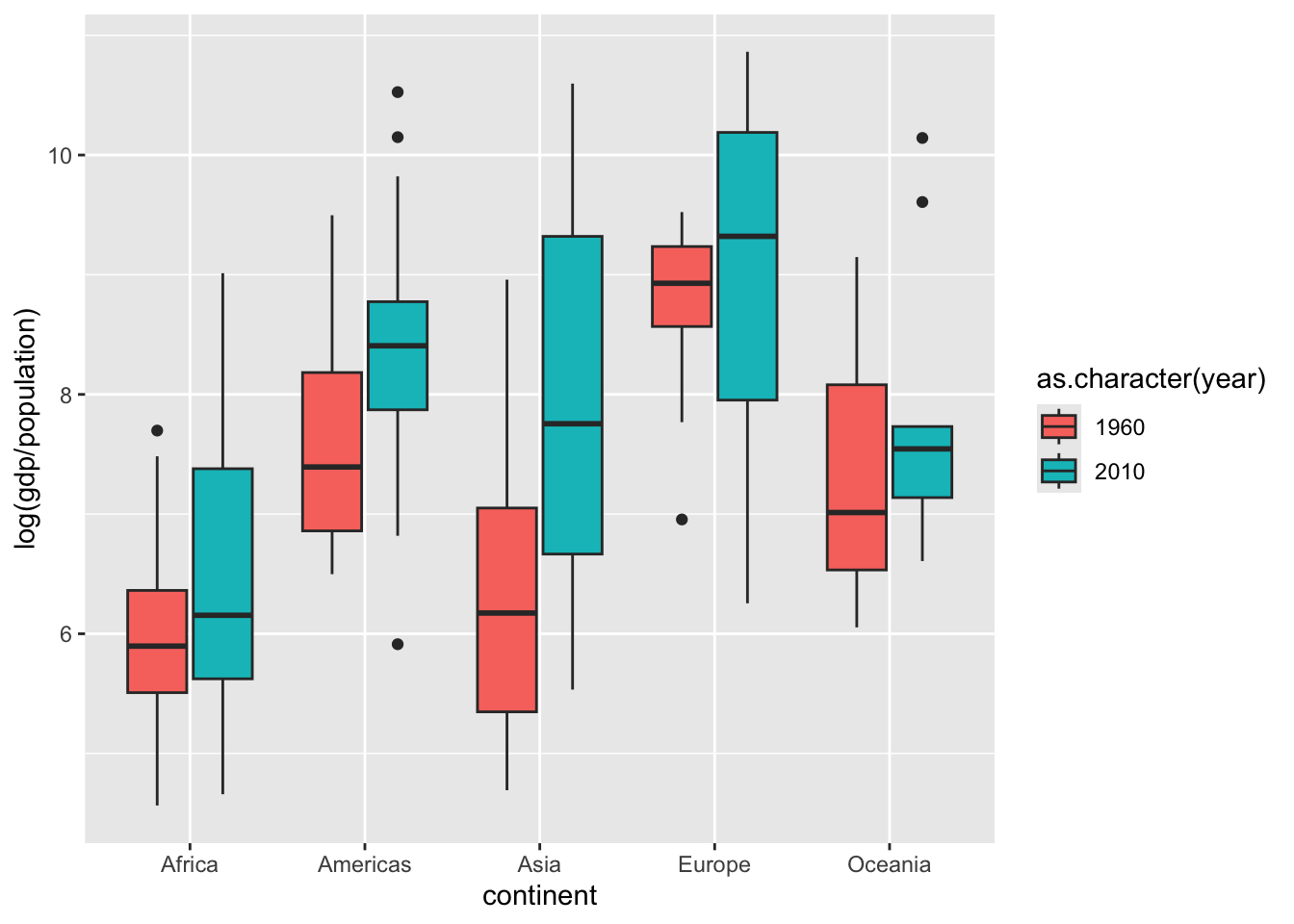## Recap (10 minutes)

Share your findings, challenges, and questions with the class.

END LAB 3